## Selina Concise Mathematics Class 10 ICSE Solutions Geometric Progression

Selina Publishers Concise Mathematics Class 10 ICSE Solutions Chapter 11 Geometric Progression

### Geometric Progression Exercise 11A – Selina Concise Mathematics Class 10 ICSE Solutions

Question 1.
Find, which of the following sequence form a G.P. :
(i) 8, 24, 72, 216, ……
(ii) $$\frac{1}{8}, \frac{1}{24}, \frac{1}{72}, \frac{1}{216}$$, ……..
(iii) 9, 12, 16, 24, ……
Solution 1(i).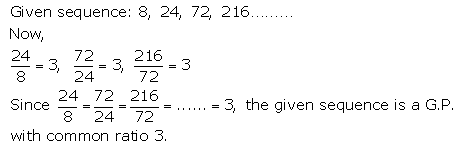Solution 1(ii).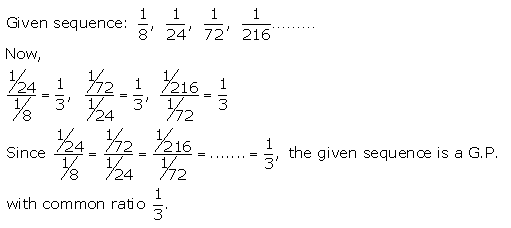Solution 1(iii).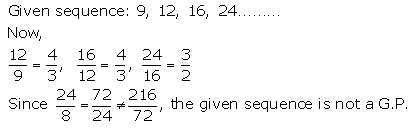Question 2.
Find the 9th term of the series :
1, 4, 16, 64 ……..
Solution: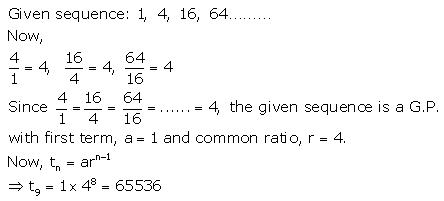Question 3.
Find the seventh term of the G.P. :
1, $$\sqrt{3}$$, 3, $$3 \sqrt{3}$$ …..
Solution:Question 4.
Find the 8th term of the sequence :
$$\frac{3}{4}, 1 \frac{1}{2}$$ 3, …….
Solution: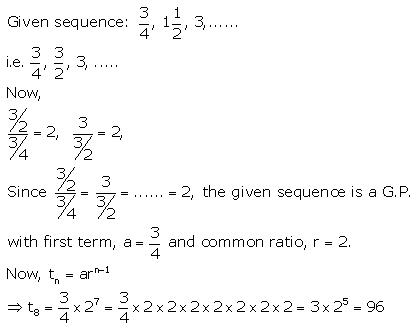Question 5.
Find the 10th term of the G.P. :
Solution:Question 6.
Find the nth term of the series :
Solution:Question 7.
Find the next three terms of the sequence :
$$\sqrt{5}$$, 5, $$5 \sqrt{5}$$, ……
Solution: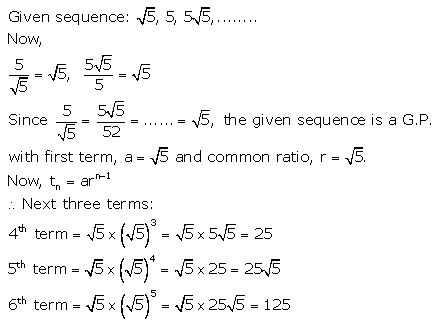Question 8.
Find the sixth term of the series :
22, 23, 24, ……….
Solution: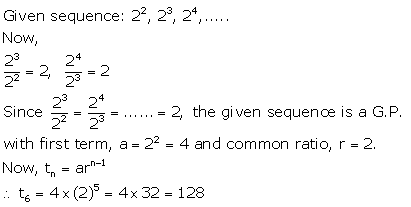Question 9.
Find the seventh term of the G.P. :
[late]\sqrt{3}+1,1, \frac{\sqrt{3}-1}{2}[/latex], ……………..
Solution: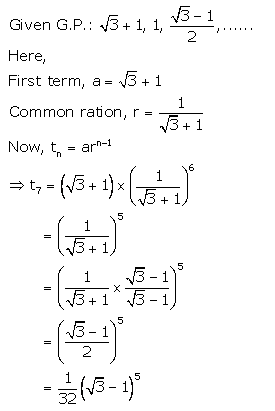Question 10.
Find the G.P. whose first term is 64 and next term is 32.
Solution:Question 11.
Find the next three terms of the series:
$$\frac{2}{27}, \frac{2}{9}, \frac{2}{3}$$, ………….
Solution: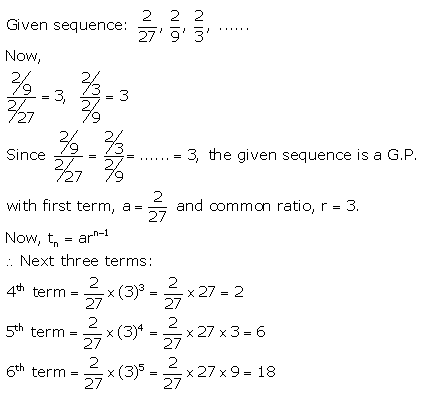Question 12.
Find the next two terms of the series
2 – 6 + 18 – 54 …………
Solution:### Geometric Progression Exercise 11B – Selina Concise Mathematics Class 10 ICSE Solutions

Question 1.
Which term of the G.P. :Solution:Question 2.
The fifth term of a G.P. is 81 and its second term is 24. Find the geometric progression.
Solution: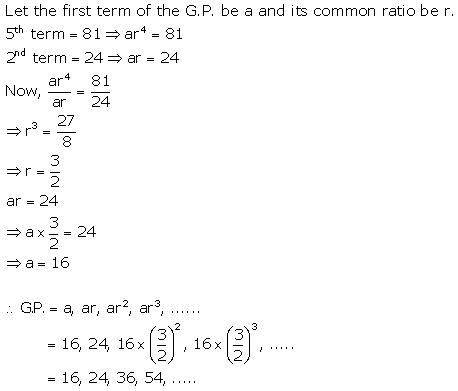Question 3.
Fourth and seventh terms of a G.P. are $$\frac{1}{18} \text { and }-\frac{1}{486}$$ respectively. Find the GP.
Solution:Question 4.
If the first and the third terms of a G.P. are 2 and 8 respectively, find its second term.
Solution: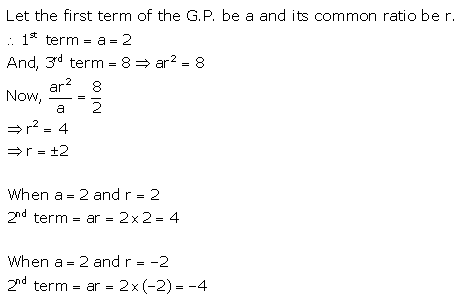Question 5.
The product of 3rd and 8th terms of a G.P. is 243. If its 4th term is 3, find its 7th term.
Solution: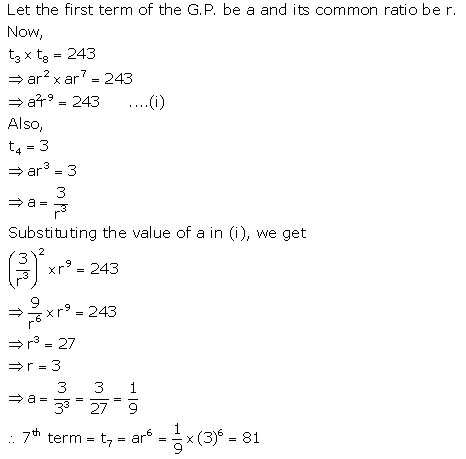Question 6.
Find the geometric progression with 4th term = 54 and 7th term = 1458.
Solution:Question 7.
Second term of a geometric progression is 6 and its fifth term is 9 times of its third term. Find the geometric progression. Consider that each term of the G.P. is positive.
Solution: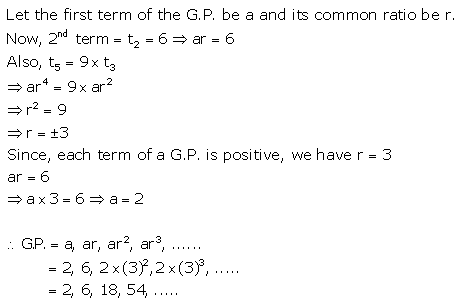Question 8.
The fourth term, the seventh term and the last term of a geometric progression are 10, 80 and 2560 respectively. Find its first term, common ratio and number of terms.
Solution:Question 9.
If the 4th and 9th terms of a G.P. are 54 and 13122 respectively, find the GP. Also, find its general term.
Solution: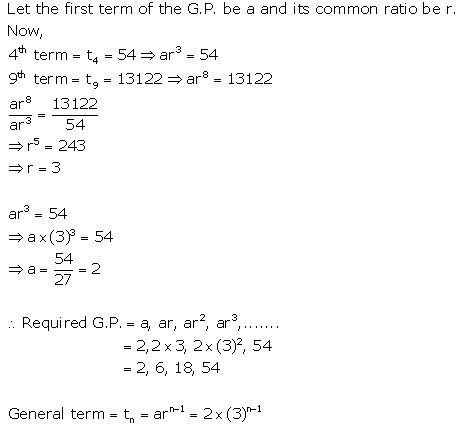Question 10.
The fifth, eight and eleventh terms of a geometric progression are p, q and r respectively. Show that : q2 = pr.
Solution: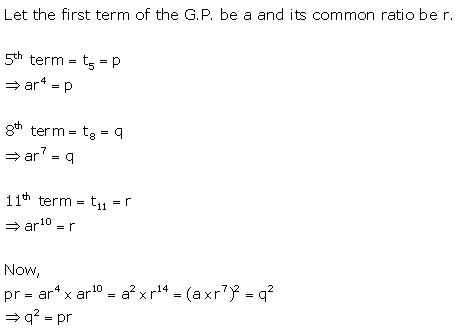### Geometric Progression Exercise 11C – Selina Concise Mathematics Class 10 ICSE Solutions

Question 1.
Find the seventh term from the end of the series : $$\sqrt{2}$$ , 2, $$2 \sqrt{2}$$, ………. 32.
Solution: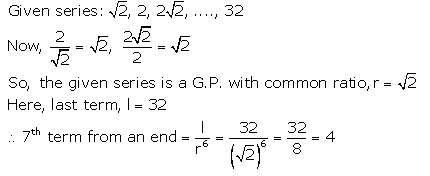Question 2.
Find the third term from the end of the GP.
$$\frac{2}{27}, \frac{2}{9}, \frac{2}{3}$$, ………….. 162
Solution:Question 3.
For the $$\frac{1}{27}, \frac{1}{9}, \frac{1}{3}$$, ………… 81;
find the product of fourth term from the beginning and the fourth term from the end.
Solution: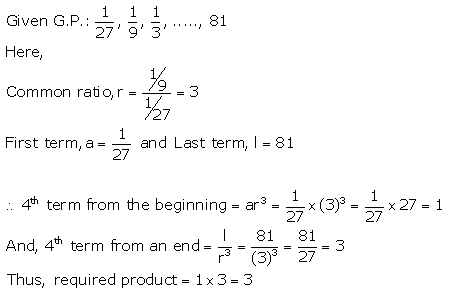Question 4.
If for a G.P., pth, qth and rth terms are a, b and c respectively ; prove that :
(q – r) log a + (r – p) log b + (p – q) log c = 0
Solution:Question 5.
If a, b and c in G.P., prove that : log an, log bn and log cn are in A.P.
Solution:Question 6.
If each term of a G.P. is raised to the power x, show that the resulting sequence is also a G.P.
Solution: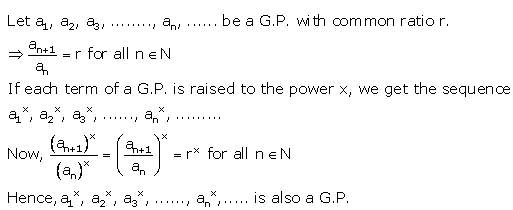Question 7.
If a, b and c are in A.P. a, x, b are in G.P. whereas b, y and c are also in G.P. Show that : x2, b2, y2 are in A.P.
Solution: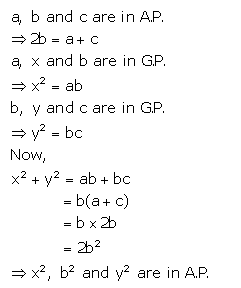Question 8.
If a, b, c are in G.P. and a, x, b, y, c are in A.P., prove that :Solution 8(i).Solution 8(ii).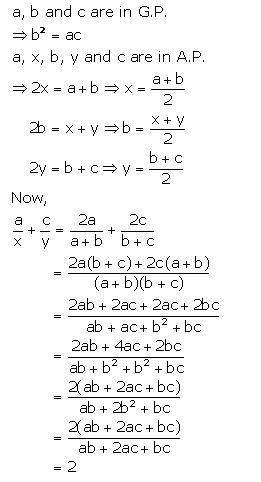Question 9.
If a, b and c are in A.P. and also in G.P., show that: a = b = c.
Solution: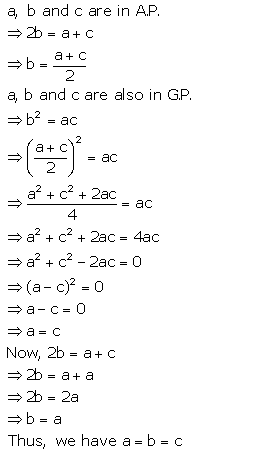Question 10.
The first term of a G.P. is a and its nth term is b, where n is an even number.If the product of first n numbers of this G.P. is P ; prove that : p2 – (ab)n.
Solution:Question 11.
If a, b, c and d are consecutive terms of a G.P. ; prove that :
(a2 + b2), (b2 + c2) and (c2 + d2) are in GP.
Solution: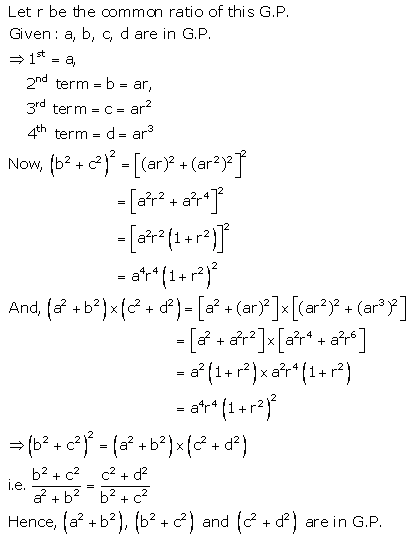Question 12.
If a, b, c and d are consecutive terms of a G.P. To prove: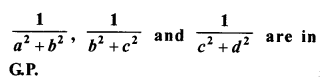Solution:### Geometric Progression Exercise 11D – Selina Concise Mathematics Class 10 ICSE Solutions

Question 1.
Find the sum of G.P. :
(i) 1 + 3 + 9 + 27 + ……….. to 12 terms.
(ii) 0.3 + 0.03 + 0.003 + 0.0003 + …… to 8 terms.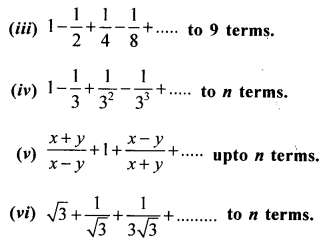Solution 1(i).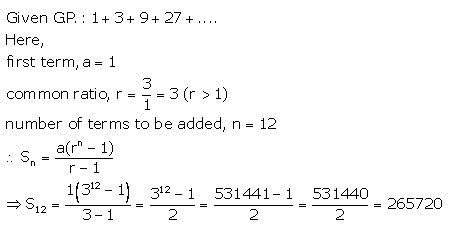Solution 1(ii).Solution 1(iii).Solution 1(iv).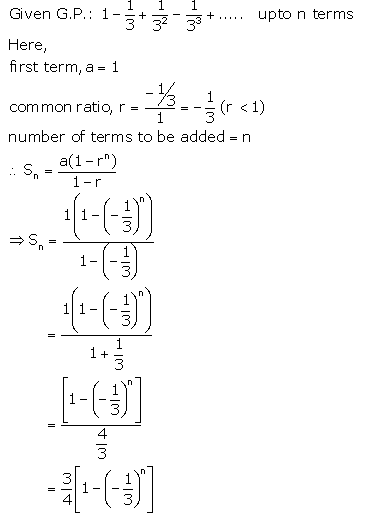Solution 1(v).Solution 1(vi).Question 2.
How many terms of the geometric progression 1+4 + 16 + 64 + ……… must be added to get sum equal to 5461?
Solution: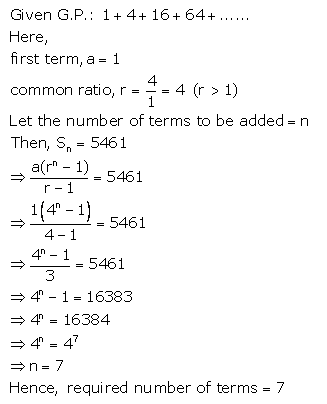Question 3.
The first term of a G.P. is 27 and its 8th term is $$\frac{1}{81}$$. Find the sum of its first 10 terms.
Solution: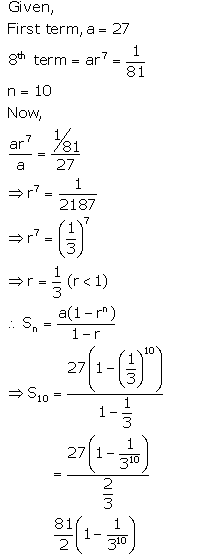Question 4.
A boy spends ₹ 10 on first day, ₹ 20 on second day, ₹ 40 on third day and so on. Find how much, in all, will he spend in 12 days?
Solution: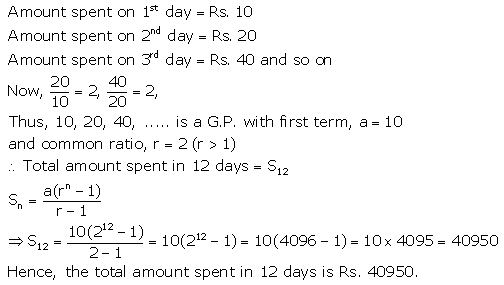Question 5.
The 4th and the 7th terms of a G.P. are $$\frac{1}{27} \text { and } \frac{1}{729}$$ respectively. Find the sum of n terms of this G.P.
Solution:Question 6.
A geometric progression has common ratio = 3 and last term = 486. If the sum of its terms is 728 ; find its first term.
Solution: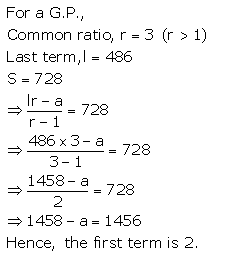Question 7.
Find the sum of G.P. : 3, 6, 12, ……………. 1536.
Solution: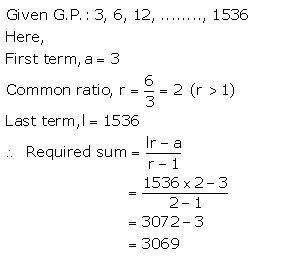Question 8.
How many terms of the series 2 + 6 + 18 + ………….. must be taken to make the sum equal to 728 ?
Solution: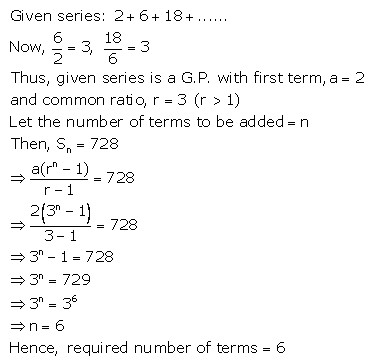Question 9.
In a G.P., the ratio between the sum of first three terms and that of the first six terms is 125 : 152.
Find its common ratio.
Solution:Question 10.
Find how many terms of G.P. $$\frac{2}{9}-\frac{1}{3}+\frac{1}{2}$$ ………. must be added to get the sum equal to $$\frac{55}{72}$$?
Solution:Question 11.
If the sum 1 + 2 + 22 + ………. + 2n-1 is 255, find the value of n.
Solution:Question 12.
Find the geometric mean between :
(i) $$\frac{4}{9} \text { and } \frac{9}{4}$$
(ii) 14 and $$\frac{7}{32}$$
(iii) 2a and 8a3
Solution 12(i).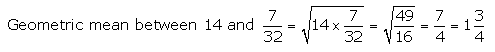Solution 12(ii).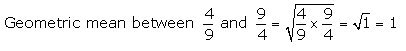Solution 12(iii).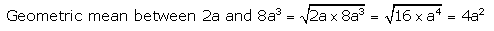Question 13.
The sum of three numbers in G.P. is $$\frac{39}{10}$$ and their product is 1. Find the numbers.
Solution:Question 14.
The first term of a G.P. is -3 and the square of the second term is equal to its 4th term. Find its 7th term.
Solution: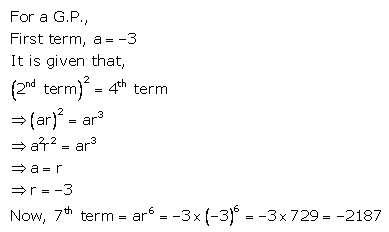Question 15.
Find the 5th term of the G.P. $$\frac{5}{2}$$, 1, …..
Solution: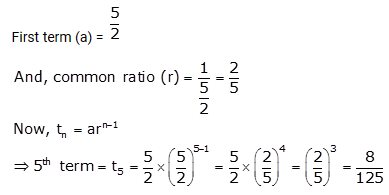Question 16.
The first two terms of a G.P. are 125 and 25 respectively. Find the 5th and the 6th terms of the G.P.
Solution: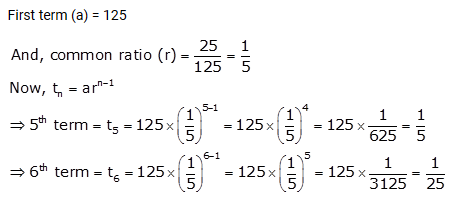Question 17.
Find the sum of the sequence –$$\frac{1}{3}$$, 1, – 3, 9, …………. upto 8 terms.
Solution: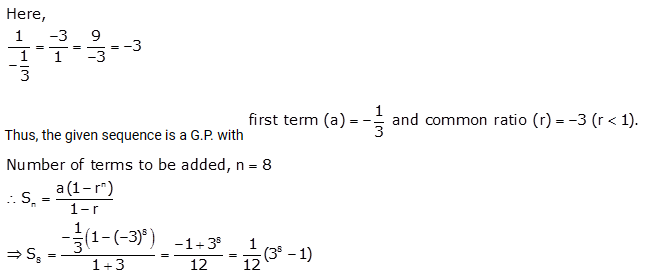Question 18.
The first term of a G.P. in 27. If the 8thterm be $$\frac{1}{81}$$, what will be the sum of 10 terms ?
Solution:Question 19.
Find a G.P. for which the sum of first two terms is -4 and the fifth term is 4 times the third term.
Solution: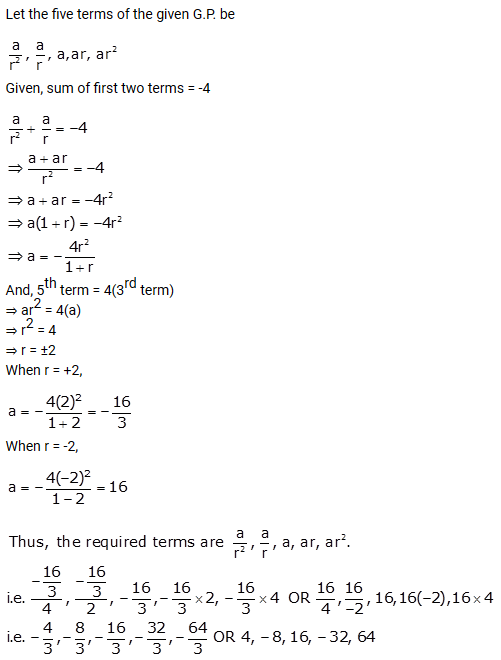Question 1.
Find the sum of n terms of the series :
(i) 4 + 44 + 444 + ………
(ii) 0.8 + 0.88 + 0.888 + …………..
Solution 1(i).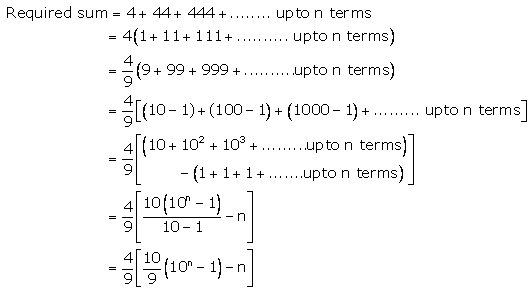Solution 1(ii).Question 2.
Find the sum of infinite terms of each of the following geometric progression: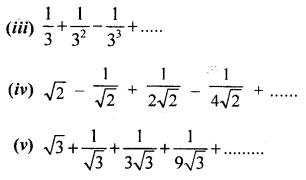Solution 2(i).Solution 2(ii).Solution 2(iii).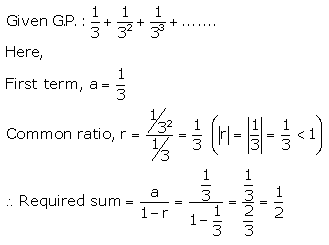Solution 2(iv).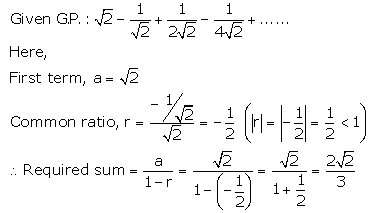Solution 2(v).Question 3.
The second term of a G.P. is 9 and sum of its infinite terms is 48. Find its first three terms.
Solution: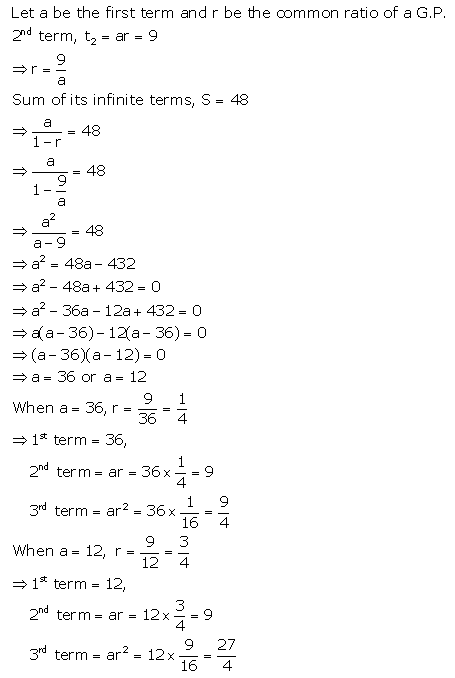Question 4.
Find three geometric means between $$\frac{1}{3}$$ and 432.
Solution:Question 5.
Find :
(i) two geometric means between 2 and 16
(ii) four geometric means between 3 and 96.
(iii) five geometric means between $$3 \frac{5}{9}$$ and $$40 \frac{1}{2}$$
Solution 5(i).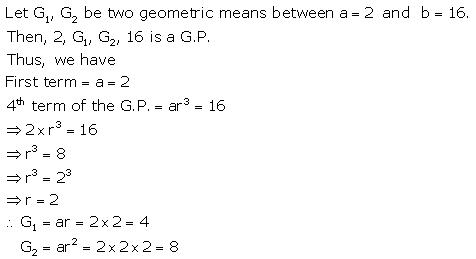Solution 5(ii).Solution 5(iii).Question 6.
The sum of three numbers in G.P. is $$\frac{39}{10}$$ and their product is 1. Find the numbers.
Solution:
Sum of three numbers in G.P. = $$\frac{39}{10}$$ and their product = 1
Let number be $$\frac{a}{r}$$, a, ar, then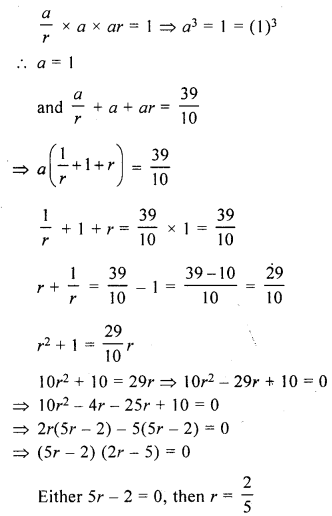Question 7.
Find the numbers in G.P. whose sum is 52 and the sum of whose product in pairs is 624.
Solution:Question 8.
The sum of three numbers in G.P. is 21 and the sum of their squares is 189. Find the numbers.
Solution:More Resources for Selina Concise Class 10 ICSE Solutions